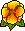## Asymptotics without logarithmic terms for crack problems

Martin Costabel, Monique Dauge, Roland DuduchavaWe consider boundary value problems for elliptic systems in a domain complementary to a smooth surface M with boundary E. The same boundary conditions are prescribed on both sides of the surface M. The most important model behind this investigation is the crack problem in three-dimensional linear elasticity (either isotropic or anisotropic): there the boundary conditions are Neumann, ie tractions are prescribed on both faces of the crack surface M. We prove that the singular functions appearing in the expansion of the solution along the crack edge E all have the form r^(k+1/2) ψ(θ) in local polar coordinates (r,θ) : The logarithmic shadow terms predicted by the general theory do not appear. Moreover, we obtain that, for a smooth right hand side, the jump of the displacement across the crack surface is the product of r^(1/2) with a smooth vector function on M.

We present two different, but complementing, approaches leading to these results, and providing distinct generalizations. The first one is based on a Wiener-Hopf factorization of the pseudodifferential symbol on the surface M obtained after reduction of the boundary value problem. The condition on the symbol which yields the absence of logarithmic terms into the solution of the boundary pseudodifferential equation is a variant of the transmission condition. The asymptotics of the solution in the full space is then deduced by a representation formula from the asymptotics of the solution on M. The second approach concerns directly the boundary value problem and is based on a closer look at the Mellin symbol at each point of the crack edge E. The Mellin symbol is proved to act between special subspaces of angular functions and the absence of logarithmic terms is the consequence of a series of compatibility conditions, valid for any Agmon--Douglis--Nirenberg system.October. 2001. Preprint IRMAR 01-50.

Comm. P.D.E. 28, no 5 & 6, 2003, 869-926.Postscript file (688 k) --- Pdf file (440 k)